The Planck Relation

The energy gap of the semiconductor in an LED is related to the threshold voltage of the LED and to the energy of light emitted by it. The relation is: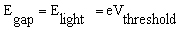(1)

where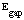is the value of the energy gap and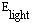is the energy of the brightest light emitted by the LED. (Although these energies are actually approximately equal to one another, we will assume they are equal.)

The energy of a photon () is related to its frequency (f) by the Planck-Einstein equation: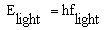(2)

where h is Planck’s constant.

The frequency of the light emitted is related to its wavelength (l) :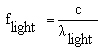(3)

where c is the speed of light (). We can write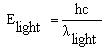(4)